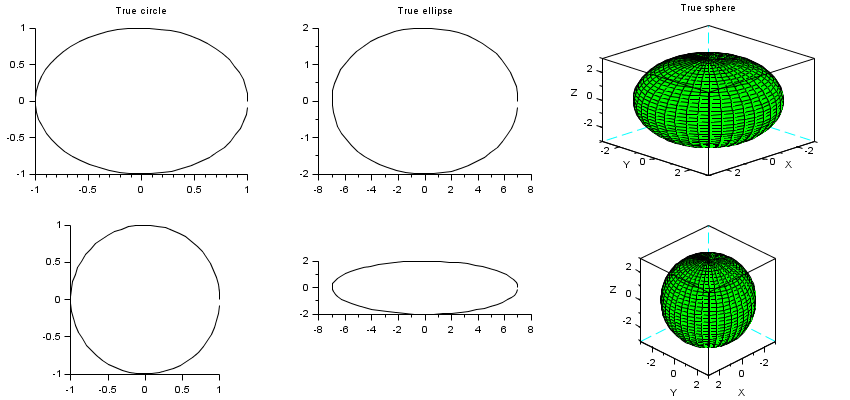Change language to:
English - Français - 日本語 - Português -

See the recommended documentation of this function

Справка Scilab >> Графики > axes_operations > isoview

# isoview

tunes isometric rendering of graphical axes

### Syntax

```isoview
isoview on
isoview off
isoview(idGraphics [,"on"|"off"])
isoview(xmin, xmax, ymin, ymax)    // DEPRECATED. use replot()```

### Arguments

"on" | "off" | <none>
Enables or disables or switches the isometric rendering for all considered axes.
idGraphics
Vector of graphic handles. All the related axes are processed. By default, the current axes is processed.
xmin, xmax, ymin, ymax
Four decimal numbers: new axes bounds.These parameters will be removed from Scilab 6.1. Please used replot after `isoview` instead.

### Description

`isoview` is used to set or relax isometric rendering scales on the x, y (and z) axes, without changing the size of the graphic window.

`isoview()` or simply `isoview` toggles the isoview status of the current axes, and keeps its current bounds: isometric scales are set whenever they were free, or scales are relaxed whenever they were isometric.

`isoview("on")` or `isoview on` sets isometric scales for the current axes.

`isoview("off")` or `isoview off` relaxes x, y (and z) scales for the current axes.

`isoview(idGraphics,..)` runs on axes related to each graphic object listed in the vector `idGraphics`:

• If `idGraphics(i)` is a figure's handle, all its Axes are considered.
• If `idGraphics(i)` is an Axes, it is considered as is.
• If `idGraphics(i)` is the handle of a sub-axes component(such as a polyline, a compound, etc), its hosting axes is considered.

`isoview(xmin, xmax, ymin, ymax)` sets the current axes in isometric mode, and then sets its bounds to the given values.This usage is deprecated and will be removed from Scilab 6.1 onwards. Please use replot after `isoview` instead.The current axes graphical entity is still the same after calling `isoview(..)`.To plot some curves directly in isometric mode without calling `isoview` afterwards, the `plot2d(...,frameflag=4)` option may be used at call time.

### Examples

```t = [0:0.1:2*%pi]';
clf
nc = 3;

subplot(2,nc,1)
plot2d(cos(t),sin(t))
xtitle("True circle")
a1 = gca();

subplot(2,nc,nc+1)
plot2d(cos(t),sin(t))
isoview

subplot(2,nc,2)
plot2d(7*cos(t),2*sin(t))
xtitle("True ellipse")
a2 = gca();

subplot(2,nc,nc+2)
plot2d(7*cos(t),2*sin(t))
isoview

// plot of a sphere using facets computed by eval3dp
deff("[x,y,z] = sph(alp,tet)",[
"x = r*cos(alp).*cos(tet)+orig(1)*ones(tet)"; ..
"y = r*cos(alp).*sin(tet)+orig(2)*ones(tet)"; ..
"z = r*sin(alp)+orig(3)*ones(tet)"]);
r = 3; orig = [0 0 0];
[xx,yy,zz] = eval3dp(sph,linspace(-%pi/2,%pi/2,40),linspace(0,%pi*2,40));
subplot(2,nc,3)
plot3d(xx, yy, zz, alpha=60, flag=[color("green") 8 4])
xtitle("True sphere")
a3 = gca();
subplot(2,nc,nc+3)
plot3d(xx, yy, zz, alpha=60, flag=[color("green") 8 4])
isoview

// Switching isoview status for all axes
isoview(gcf())

// Forcing isoview for all axes of the current figure
isoview(gcf(), "on")

// Relaxing isoview for the current axes
isoview off

// Setting isoview for top axes
isoview(gcf(),"off")
isoview([a1 a2 a3], "on")``````// Using plot2d(..., frameflag=4) :
r = linspace(1,10,200);
t = r*3;
scf();
subplot(1,2,1)
plot2d(r.*sin(t),r.*cos(t))
xtitle("plot2d(..)")

subplot(1,2,2)
plot2d(r.*sin(t),r.*cos(t),frameflag=4)
xtitle("with frameflag=4")```Version Description 6.0 input flags "on"|"off" added. input parameters or idGraphics added. `isoview(xmin, xmax, ymin, ymax)` marked as deprecated.